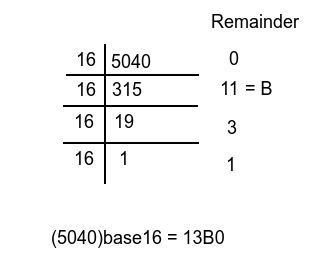# Find the Number of Trailing Zeroes in Base 16 Representation of N! using C++

In this article, we will understand the problem of finding trailing zeros of a given number N in the base 16 representation of its factorial for example

Input : N = 7
Output : 1
Explanation : fact(7) = 5040 in base10 and 13B0 in base16 having 1 trailing zero.

Input : N = 11
Output : 2
Explanation : fact(11) = 39916800 in base10 and 2611500 in base16 having 2 trailing zeroes.

Let's first recap the process of converting any decimal number from one base to another; let's take an example of converting (5040)10 to (?)16i.e., dividing the number by 16 and keeping the remainder until the number cannot be further divided. The result will be the remainder in reverse order.

As a result, we have one trailing zero, and this trailing zero we got when 16 divides the number with remainder 0.

Prime factorization of 5040 = 161 * 451* 71, which means 16 divides 5040 1 time with remainder 0, equal to trailing zeroes. In this way, we can calculate the number of trailing zeroes.

## Approach to find The Solution

We discussed above the way to find the number of trailing zeroes. We also know 16 = 24, which means the highest power 2 in N divided by four will be equal to the highest power of 16. It is also known as Legendre's formula.

## C++ Code for the Above Approach

Here is the C++ syntax which we can use as an input to solve the given problem −

## Example

#include <bits/stdc++.h>
using namespace std;

int main () {
int n = 11;
long long int count = 0;
long long int value, power = 2;
long long int result;

do{
value = n / power;
count += value;
power *= 2;
}
while (value != 0);

// Count has the highest power of 2
result = count / 4;
cout << "Number of trailing zeroes in base 16 representation of N : " << result;
}

## Output

Number of trailing zeroes in base 16 representation of N: 2

## Explanation of the above code

• We are initializing power with two as we need to calculate the highest power 2.
• Implementing Legendre’s formula in a while loop where we divide n with the power, which is initially two, and incrementing count with the value and power with 2.
• After dividing the highest power of 2 with four which is stored in the count variable.
• We are finally printing the result.

## Conclusion

In this article, we solve the problem of finding the number of trailing zeroes in the base16 representation of factorial N, which we solve using Legendre’s formula. We also write C++ code to solve the same problem. You can write this code in any other language like Java, C, python, etc. We hope you find this article helpful.

Updated on: 25-Nov-2021

101 Views